﻿ Graphing Hyperbolas

# Graphing Hyperbolas

LESSON READ-THROUGH (Part 1 of 2)
by Dr. Carol JVF Burns (website creator)
Follow along with the highlighted text while you listen!
• PRACTICE (online exercises and printable worksheets)

Hyperbolas were introduced in three prior lessons:

The purpose of this current section is to go from the equation of a hyperbola to its graph.
In particular, we'll see that every hyperbola has a so-called central box that is helpful in determining its shape.

Short on time? Jump right to the summary!

This lesson includes lots of details.
Once you understand things, it can be written more compactly.

For your convenience, the standard equations of hyperbolas
(that were derived in the preceding section) are repeated here:

## Equations of Hyperbolas: Center at the Origin

In both equations, $\,a > 0\,$ and $\,b > 0\,.$
Also, $\,c\,$ is the positive number for which $\,c^2 = a^2 + b^2\,.$
With the equations in standard form, $\,a^2\,$ is the denominator of the positive term on the left side.

## Foci on the $x$-axis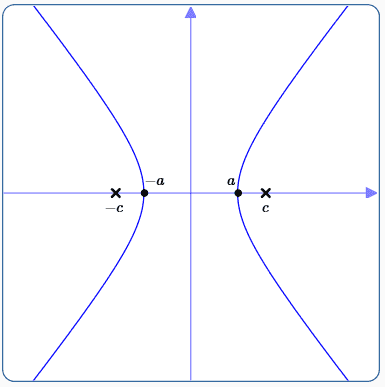$$\cssId{s17}{\frac{x^2}{a^2} - \frac{y^2}{b^2} = 1}$$ Vertices: $\,(-a,0)\,$ and $\,(a,0)\,$
Foci: $\,(-c,0)\,$ and $\,(c,0)\,$

## Foci on the $y$-axis$$\cssId{s21}{\frac{y^2}{a^2} - \frac{x^2}{b^2} = 1}$$ Vertices: $\,(0,-a)\,$ and $\,(0,a)\,$
Foci: $\,(0,-c)\,$ and $\,(0,c)\,$

## Review:

The following facts are used in this lesson:

• The square root function is increasing:
In particular, if $\,0 \le x \le y\,,$ then $\,0 \le \sqrt{x} \le \sqrt{y}\,.$
• Renaming the square root of a square:
For all real numbers $\,x\,,$ $\sqrt{x^2} = |x|\,.$
• The absolute value of a nonnegative number is itself:
If $\,x \ge 0\,,$ then $\,|x| = x\,.$
• Squared quantities are nonnegative:
For all real numbers $\,x\,,$ $\,x^2 \ge 0\,.$
• Solving an absolute value inequality:
For all real numbers $\,x\,$ and for $\,k > 0\,$: $$\cssId{s37}{|x| \ge k\ \ \ \ \text{is equivalent to}\ \ \ \ (x\ge k\ \ \text{ or }\ \ x\le -k)}$$
• The square root of a product is the product of the square roots:
Providing both $\,x\ge 0\,$ and $\,y\ge 0\,,$ then $\,\sqrt{xy} = \sqrt{x}\sqrt{y}\,.$
• Solving an absolute value equation:
For all real numbers $\,x\,$ and for $\,k \ge 0\,$: $$\cssId{s42}{|x| = k\ \ \ \ \text{is equivalent to}\ \ \ \ x = \pm k}$$

## Important Information Given by the Equations of Hyperbolas

Many equations easily give you lots of information about their graphs, without any memorizing!
This is certainly true with hyperbolas, as follows.

## What part of the plane do hyperbolas live in?

Let's rewrite and analyze the equation $\,\displaystyle \frac{x^2}{a^2} - \frac{y^2}{b^2} = 1\,$:

\displaystyle \begin{alignat}{2} \frac{x^2}{a^2} &= 1 + \frac{y^2}{b^2} &\qquad&\text{(solve for the term containing\,x\,$)}\cr &\ge 1 &&\text{(since$\,\frac{y^2}{b^2} \ge 0\,)} \end{alignat}

Thus:

\displaystyle \begin{alignat}{2} &\cssId{s50}{\frac{x^2}{a^2}\ge 1}&&\cr\cr &\cssId{s51}{x^2\ge a^2} &\qquad&\cssId{s52}{\text{(multiply both sides by\,a^2 > 0\,)}} \end{alignat}

Take note of this intermediate result:
it follows that for any point on the hyperbola, $\,x^2 - a^2 \ge 0\,.$
We'll use this fact below.

Continuing:

\displaystyle \begin{alignat}{2} &\cssId{s57}{\sqrt{\strut x^2} \ge \sqrt{\strut a^2}}&&\cssId{s58}{\text{(the square root function is increasing)}}\cr\cr &\cssId{s59}{|x| \ge |a|}&&\cssId{s60}{\text{(\,\sqrt{x^2} = |x|\,$)}}\cr\cr &\cssId{s61}{|x| \ge a}&&\cssId{s62}{\text{(since$\,a > 0\,,\,|a| = a\,)}}\cr\cr &\cssId{s63}{x \ge a\ \ \ \text{ or }\ \ \ x\le -a}&\qquad&\cssId{s64}{\text{(solving the absolute value inequality)}} \end{alignat}

This confirms that any point $\,(x,y)\,$ satisfying $\,\displaystyle\frac{x^2}{a^2} - \frac{y^2}{b^2} = 1\,$
must lie in one of the two half-planes, $\,x \ge a\,$ or $\,x\le -a\,,$ shaded green below: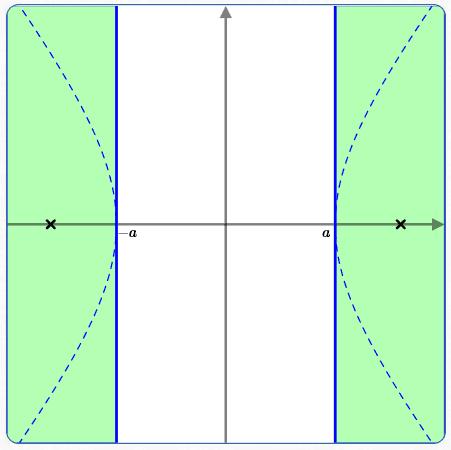## What do hyperbolas look like when $\,x\,$ is big?

What happens to the graph of   $\,\displaystyle\frac{x^2}{a^2} - \frac{y^2}{b^2} = 1\,$   for large values of $\,x\,$?
To answer this question, first ‘re-name’ the equation by solving for $\,y\,$:

\begin{alignat}{2} &\cssId{s69}{\frac{y^2}{b^2} = \frac{x^2}{a^2} - 1}&\qquad&\cssId{s70}{\text{(re-arrange)}}\cr\cr &\cssId{s71}{y^2 = b^2\left(\frac{x^2}{a^2} - 1\right)}&&\cssId{s72}{\text{(multiply both sides by \,b^2\,)}}\cr\cr &\cssId{s73}{y^2 = b^2\left(\frac{x^2}{a^2} - \frac{a^2}{a^2}\right)}&&\cssId{s74}{\text{(rename \,1\, as \,\frac{a^2}{a^2})}}\cr\cr &\cssId{s75}{y^2 = \frac{b^2}{a^2}(x^2 - a^2)}&&\cssId{s76}{\text{(factor out \,\frac{1}{a^2})}}\cr\cr &\cssId{s77}{\sqrt{y^2} = \sqrt{\frac{b^2}{a^2}(x^2 - a^2)}}&\qquad&\cssId{s78}{\text{(take square roots of both sides)}}\cr\cr &\cssId{s79}{|y| = \sqrt{\frac{b^2}{a^2}(x^2 - a^2)}}&\qquad&\cssId{s80}{\text{(\sqrt{y^2} = |y|\,)}}\cr\cr &\cssId{s81}{|y| = \sqrt{\frac{b^2}{a^2}} \sqrt{\strut x^2 - a^2}}&\qquad&\cssId{s82}{\text{(as shown above, \,x^2 - a^2\ge 0\, for all points on the hyperbola)}}\cr\cr &\cssId{s83}{|y| = \frac{b}{a}\sqrt{\strut x^2 - a^2}}&&\cssId{s84}{\text{(both \,a > 0\, and \,b > 0\,)}}\cr\cr &\cssId{s85}{|y| = \frac{b}{a}\sqrt{\strut x^2\bigl(1 - \frac{a^2}{x^2}\bigr)}}&&\cssId{s86}{\text{(factor out \,x^2\,; we're exploring this equation for BIG \,x\, right now, so \,x\ne 0\,)}}\cr\cr &\cssId{s87}{y = \pm\frac{b}{a}\sqrt{\strut x^2\bigl(1 - \frac{a^2}{x^2}\bigr)}}&&\cssId{s88}{\text{(solve the absolute value equation; notice that the prior right-hand side is nonnegative)}}\cr\cr \end{alignat}

This version of the equation might look harder than what we started with ($\displaystyle\frac{x^2}{a^2} - \frac{y^2}{b^2} = 1$),
but it's better for understanding what happens when $\,x\,$ gets big.

Here's the key idea (which will be firmed up in Calculus):

As $\,x\,$ gets bigger and bigger, $\,\displaystyle\frac{a^2}{x^2}\,$ looks more and more like $\,0\,.$

More precisely:

We can get $\,\displaystyle\frac{a^2}{x^2}\,$ as close to $\,0\,$ as we want,
by making $\,x\,$ sufficiently large.

As an example, let $\,x = 1000a\,.$
(Here, $\,x\,$ isn't particularly big—it's just one thousand times bigger than $\,a\,.$ )
Already, though: $$\cssId{s100}{\frac{a^2}{x^2} = \frac{a^2}{(1000a)^2} = \frac{1}{1{,}000{,}000}}$$ That's pretty close to zero!

So, here's what happens to the equation   $\displaystyle\,y = \pm\frac{b}{a}\sqrt{\strut x^2\bigl(1 - \frac{a^2}{x^2}\bigr)}\,$   as $\,x\,$ gets big (big positive, or big negative):

• As $\,x\,$ gets big, $\,\displaystyle\frac{a^2}{x^2}\,$ approaches $\,0\,.$
• As $\,\displaystyle\frac{a^2}{x^2}\,$ approaches $\,0\,,$   $\,\displaystyle(1-\frac{a^2}{x^2})\,$ approaches $\,1\,.$
• As $\,\displaystyle(1-\frac{a^2}{x^2})\,$ approaches $\,1\,,$ the expression $\displaystyle\,x^2(1-\frac{a^2}{x^2})\,$ looks more and more like $\,x^2\,.$
• Thus,   $\,y = \pm\frac{b}{a}\sqrt{\strut x^2\bigl(1 - \frac{a^2}{x^2}\bigr)}\,$   looks more and more like $\,y = \pm\frac{b}{a}\sqrt{x^2}\,,$ which simplifies to $\,y = \pm\frac{b}{a}|x|\,.$
• This cleans up even more!
Because of the ‘plus or minus’ sign, we can lose the absolute value.
Here are the details:
• If $\,x\ge 0\,,$ then $\,|x| = x\,.$
In this case, $\,y = \pm \frac{b}{a}|x|\,$ becomes $\,y = \pm \frac{b}{a}x\,.$
• If $\,x < 0\,,$ then $\,|x| = -x\,.$
In this case, $\,y = \pm \frac{b}{a}|x|\,$ becomes $\,y = \pm \frac{b}{a}(-x) = \mp \frac{b}{a}x\,.$
• ‘$\displaystyle\,y = \pm \frac{b}{a}x\,$’ is a shorthand for ‘$\,y = \frac bax \ \text{ or } \ y = -\frac bax\,$’.

‘$\displaystyle\,y = \mp \frac{b}{a}x\,$’ is a shorthand for ‘$\,y = -\frac bax \ \text{ or }\ y = \frac bax\,$’.
• The sentence ‘$\,A \text{ or } B\,$’   is equivalent to   ‘$\,B\text{ or } A\,$’.
• Thus, $\,y = \pm \frac{b}{a}|x|\,$ is equivalent to $\,y = \pm\frac bax\,.$

## Asymptotes for Hyperbolas

 The lines $\displaystyle\,y = \pm\frac bax\,$ are asymptotes for the hyperbola $\displaystyle\,\frac{x^2}{a^2} - \frac{y^2}{b^2} = 1\,$. As $\,x\,$ gets big (positive or negative), the graph of $\displaystyle\,\frac{x^2}{a^2} - \frac{y^2}{b^2} = 1\,$ gets closer and closer to these lines, as shown at right. The asymptotes form an ‘envelope’ inside which the hyperbola lives. The envelope gets tighter and tighter as $\,x\,$ gets bigger and bigger! The green box is called the central box of the hyperbola, and is a useful graphing aid: its sides are parallel to the axes it passes through the vertices of the hyperbola it crosses the other axis at $\,\pm b\,$ The diagonals of the central box are the asymptotes for the hyperbola!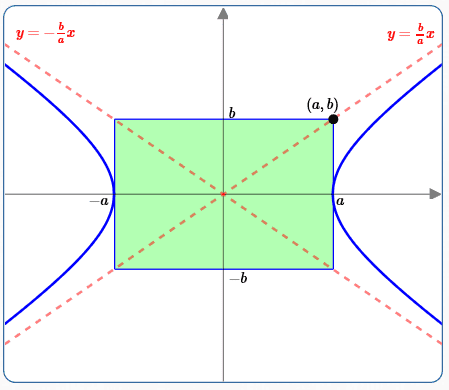Similarly, the lines $\displaystyle\,y = \pm\frac abx\,$ are asymptotes for the hyperbola $\displaystyle\,\frac{y^2}{a^2} - \frac{x^2}{b^2} = 1\,$: Compare $\displaystyle\,\frac{y^2}{a^2} - \frac{x^2}{b^2} = 1\,$ with the previously-discussed equation $\displaystyle\,\frac{x^2}{a^2} - \frac{y^2}{b^2} = 1\,$: the variables $\,x\,$ and $\,y\,$ have just been switched. Thus, the exact same derivation as before (with variables switched) yields the asymptotes $\displaystyle\,x = \pm \frac ba y\,.$ Solve for $\,y\,$ to get the asymptotes $\displaystyle\,y = \pm\frac ab x\,$. Alternatively, solve for $\,y\,$ in $\displaystyle\,\frac{y^2}{a^2} - \frac{x^2}{b^2} = 1\,$ to get: $\displaystyle\,y = \pm \frac ab\sqrt{\strut x^2\bigl(1 + \frac{b^2}{x^2}\bigr)}$ For large $\,x\,,$ the graph looks like:   $\displaystyle\,y = \pm \frac ab x\,$

## Getting the Central Box Graphically

 If you have a hyperbola graphed with the foci already located, then it's easy to get the central box graphically: Make sure that ‘$\,1\,$’ on $x$-axis is the same as ‘$\,1\,$’ on the $y$-axis (so that a circle actually looks like a circle). Sweep out (part of) the circle with center at the origin and radius $\,c\,,$ as shown at right. Mark where the circle intersects the vertical line through the vertex. The green triangle has hypotenuse $\,c\,$ and bottom leg $\,a\,.$ Since $\,c^2 = a^2 + b^2\,,$ the remaining leg must have length $\,b\,.$ Of course, you can use this information in a different way: if you already have $\,a\,$ and $\,b\,$ marked, then just rotate the hypotenuse to locate the focus!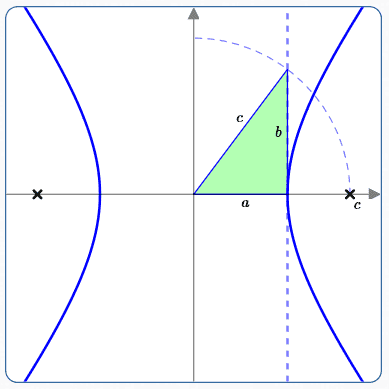## Summary: Graphing Hyperbolas$$\cssId{sb33}{\color{green}{\frac{x^2}{a^2}} - \color{red}{\frac{y^2}{b^2}} = 1}$$ Vertices: Set $\,y = 0\,,$ solve for $\,x\,,$ to get $\,x = \pm a\,.$ The positive term ($\displaystyle\color{green}{\frac{x^2}{a^2}}\,$) determines the vertices!$$\cssId{sb37}{\color{green}{\frac{y^2}{a^2}} - \color{red}{\frac{x^2}{b^2}} = 1}$$ Vertices: Set $\,x = 0\,,$ solve for $\,y\,,$ to get $\,y = \pm a\,.$ The positive term ($\displaystyle\color{green}{\frac{y^2}{a^2}}\,$) determines the vertices! Use the other term to find $\,b\,$; draw in the central box. The diagonals of the central box are the asymptotes for the hyperbola.

## Steps to Graph a Hyperbola:

• [Vertices] Find the vertices.
• [Central Box] Use the other term to find $\,b\,$; draw in the central box. (See the examples below.)
• [Diagonals] The diagonals of the central box are the asymptotes of the hyperbola.
• [Draw Hyperbola] Draw in the hyperbola, using the asymptotes as an ‘envelope’.
• [Foci] If needed, use $\,c^2 = a^2 + b^2\,$ to locate the foci.

## Examples: Graphing Hyperbolas

In both examples below, there are only $\,x^2\,,$ $\,y^2\,,$ and constant terms.
When the variable terms are on the same side, they have different signs.
So, we know we're dealing with hyperbolas!

## Example #1: Put the Equation in Standard Form

Graph: $\,9x^2 - 4y^2 = 36\,$
Find the vertices, central box, asymptotes, and foci.

When all the numbers ‘work out nicely’, it may be easiest to put the equation in standard form.
Here, both $\,9\,$ and $\,4\,$ go into $\,36\,$ evenly (resulting in perfect square denominators).

 Put the equation in standard form: \displaystyle \begin{alignat}{2} &\cssId{sb67}{\frac{9x^2}{36} - \frac{4y^2}{36} = \frac{36}{36}}&\qquad&\cssId{sb68}{\text{(get a\,1\,on the right-hand side)}}\cr\cr &\cssId{sb69}{\frac{x^2}{4} - \color{red}{\frac{y^2}{9}} = 1}&&\cssId{sb70}{\text{(simplify)}}\cr \end{alignat} (This is an equation of the form $\displaystyle\,\frac{x^2}{a^2} - \color{red}{\frac{y^2}{b^2}} = 1\,.$ ) Find the vertices: Set $\,y = 0\,$:   $\displaystyle \frac{x^2}{4} = 1\,,$   $\displaystyle x^2 = 4\,,$   $\displaystyle x = \pm 2$ Use the other term to find $\,b\,.$ Draw the central box, its diagonals, and the hyperbola: Compare $\displaystyle\,\frac{y^2}{b^2}\,$ with $\displaystyle\,\frac{y^2}{9}\,$ to see that $\,b = 3\,.$ Mark $\,\pm 3\,$ on the $y$-axis. Sketch in the central box and its diagonals (which are the asymptotes). Sketch the hyperbola inside the ‘envelope’ formed by the diagonals. Foci: We have $\,a = 2\,$ and $\,b = 3\,$:   $c^2 = a^2 + b^2 = 4 + 9 = 13\,,$   $\,c = \sqrt{13} \approx 3.6\,$ Report results: vertices:   $\,(\pm 2,0)\,$ central box:   has corners $\,(\pm 2,\pm 3)\,$ asymptotes:   $\,y = \pm \frac 32x\,$ foci:   $\,(\pm\sqrt{13},0)\,$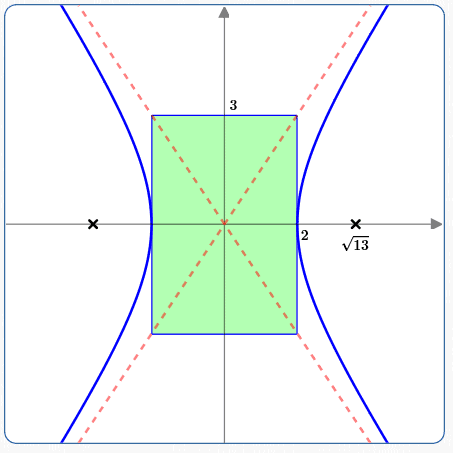You can also head up to WolframAlpha and type in:   graph 9x^2 - 4y^2 = 36
You'll get everything: graph, vertices, foci, asymptotes, and more!

## Example #2: Don't Put the Equation (Completely) in Standard Form

If the numbers don't work out nicely, then you don't have to write it completely in standard form.
As long as you get the variable terms on the left, and the ‘$\,1\,$’ on the right, you're good to go!

Graph: $\,9y^2 = 7 + 14x^2\,$
Find the vertices, central box, asymptotes, and foci.

 Get the variable terms on the left, ‘$\,1\,$’ on the right: \displaystyle \begin{alignat}{2} &\cssId{sb100}{9y^2 - 14x^2 = 7}&\qquad&\cssId{sb101}{\text{variable terms on left, constant on right}}\cr\cr &\cssId{sb102}{\frac{9y^2}{7} - \frac{14x^2}{7} = \frac{7}{7}}&\qquad&\cssId{sb103}{\text{(get a\,1\,on the right-hand side)}}\cr\cr &\cssId{sb104}{\frac{9y^2}{7} - \color{red}{2x^2} = 1} \end{alignat} (This is an equation of the form $\displaystyle\,\frac{y^2}{a^2} - \color{red}{\frac{x^2}{b^2}} = 1\,.$ ) To go from here to ‘complete’ standard form would be a bit ugly, resulting in: $\displaystyle\frac{y^2}{7/9} - \frac{x^2}{1/2} = 1$ As you'll see, this isn't needed! Find the vertices: Set $\,x = 0\,$:   $\displaystyle \frac{9y^2}{7} = 1\,,$   $\displaystyle y^2 = \frac{7}{9}\,,$   $\displaystyle y = \pm \sqrt{\frac79} = \pm\frac{\sqrt 7}3 \approx \pm 0.88$ So:   $\displaystyle \,a = \frac{\sqrt 7}{3}$ Use the other term to find $\,b\,.$ Draw the central box, its diagonals, and the hyperbola: Compare $\displaystyle\,\frac{x^2}{b^2}\,$ with $\,2x^2\,$ to find $\,b\,$: \displaystyle \begin{alignat}{2} &\cssId{sb118}{\frac{x^2}{b^2} = 2x^2}&\qquad&\cssId{sb119}{\text{(compare the term to the desired form)}}\cr\cr &\cssId{sb120}{\frac{1}{b^2} = 2}&&\cssId{sb121}{\text{(cancel\,x^2\,$)}}\cr\cr &\cssId{sb122}{b^2 = \frac 12}&&\cssId{sb123}{\text{(solve for$\,b^2\,)}}\cr\cr &\cssId{sb124}{b = \pm \frac{1}{\sqrt 2} \approx \pm 0.71} \end{alignat} Mark $\displaystyle\,\pm \frac{1}{\sqrt 2}\,$ on the $x$-axis. Sketch in the central box and its diagonals (which are the asymptotes). Sketch the hyperbola inside the ‘envelope’ formed by the diagonals. Foci: We have $\displaystyle\,a = \frac{\sqrt 7}{3}\,$ and $\displaystyle\,b = \frac{1}{\sqrt 2}\,$: $\displaystyle \cssId{sb130}{c^2 = a^2 + b^2} \cssId{sb131}{= \bigl(\frac{\sqrt 7}{3}\bigr)^2 + \bigl(\frac{1}{\sqrt 2}\bigr)^2} \cssId{sb132}{= \frac 79 + \frac 12} \cssId{sb133}{= \frac{14}{18} + \frac{9}{18}} \cssId{sb134}{= \frac{23}{18}}\,$ $\displaystyle c = \frac{\sqrt{23}}{3\sqrt{2}} \approx 1.13$ Report results: Note that: $\displaystyle \cssId{sb138}{\frac ab \ \ =\ \ \frac{\frac{\sqrt 7}{3}}{\frac{1}{\sqrt 2}}} \cssId{sb139}{\ \ =\ \ \frac{\sqrt 7}{3}\cdot \frac{\sqrt 2}{1}} \cssId{sb140}{\ \ =\ \ \frac{\sqrt{14}}{3}}$ vertices:   $\displaystyle\,\left(0 \ ,\ \pm \frac{\sqrt{7}}3\right)\,$ central box:   has corners $\displaystyle\,\left(\pm \frac{1}{\sqrt{2}} \ ,\ \pm \frac{\sqrt{7}}{3}\right)\,$ asymptotes:   $\displaystyle\,y = \pm \frac{\sqrt{14}}{3}x\,$ foci:   $\displaystyle\,\left(0 \ ,\ \pm\frac{\sqrt{23}}{3\sqrt 2}\right)\,$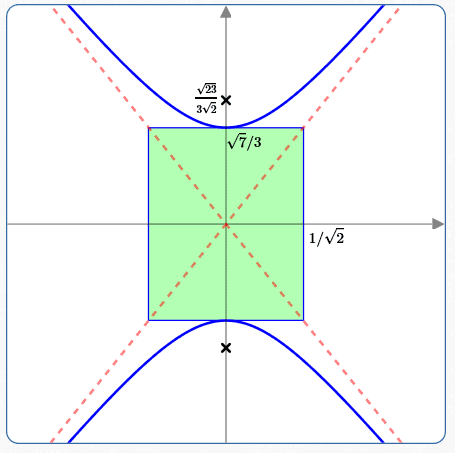Master the ideas from this section